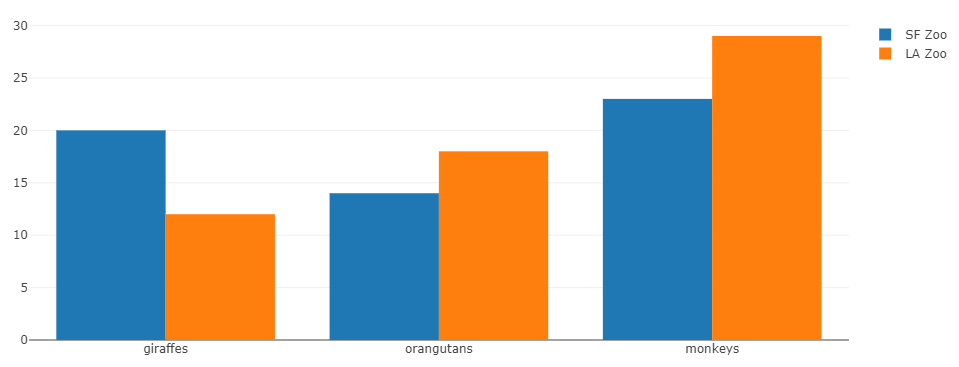# Graph Function API

In the environment panel, with a right click on a vector, you can display a simple graph, a density graph (R only), an histogram or a pie chart (R only, if the data are not numeric). It calls a function `graph()`. This function can be used independently, without any additionnal package. It uses the JavaScript API of plotly, but as an R list or a Python dictionary. There is no need to know JavaScript, at any level.

The easiest way to understand how it works is to browse a basic example. In order to display the following code from the plotly documentation, the code should be:

``````trace1 = list(                                   #var trace1 = {
x = c('giraffes', 'orangutans', 'monkeys'),  #  x: ['giraffes', 'orangutans', 'monkeys'],
y = c(20, 14, 23),                           #  y: [20, 14, 23],
name = 'SF Zoo',                             #  name: 'SF Zoo',
type = 'bar'                                 #  type: 'bar'
)                                                #};
trace2 = list(                                   #var trace2 = {
x = c('giraffes', 'orangutans', 'monkeys'),  #  x: ['giraffes', 'orangutans', 'monkeys'],
y = c(12, 18, 29),                           #  y: [12, 18, 29],
name = 'LA Zoo',                             #  name: 'LA Zoo',
type = 'bar'                                 #  type: 'bar'
)                                                #};
data = list(trace1, trace2)                      #var data = [trace1, trace2];
layout = list(barmod = 'group')                  #var layout = {barmode: 'group'};
mygraph <- list(data = data, layout = layout)    #Plotly.newPlot('myDiv', data, layout);
graph(mygraph)
``````
``````trace1 = {                                        #var trace1 = {
'x' : ['giraffes', 'orangutans', 'monkeys'],  #  x: ['giraffes', 'orangutans', 'monkeys'],
'y' : [20, 14, 23],                           #  y: [20, 14, 23],
'name' : 'SF Zoo',                            #  name: 'SF Zoo',
'type' : 'bar'                                #  type: 'bar'
}                                                 #};
trace2 = {                                        #var trace2 = {
'x' : ['giraffes', 'orangutans', 'monkeys'],  #  x: ['giraffes', 'orangutans', 'monkeys'],
'y' : [12, 18, 29],                           #  y: [12, 18, 29],
'name' : 'LA Zoo',                            #  name: 'LA Zoo',
'type' : 'bar'                                #  type: 'bar'
}                                                 #};
data = [trace1, trace2]                           #var data = [trace1, trace2];
layout = {'barmod' : 'group'}                     #var layout = {barmode: 'group'};
mygraph = {'data' : data, 'layout' : layout}      #Plotly.newPlot('myDiv', data, layout);
graph(mygraph)
``````On the left there is the R / Python code, and on the right in comment the JavaScript code from the plotly example.

Explanation:

• In the JavaScript, trace1 and trace2 are lists with named elements x, y, name, type, where x and y and are vectors/lists. We create the same objects in R / Python, as R vectors/lists or Python numpy array/dictionaries/lists.
• `data` is an R / Python list with the previous ones.
• `layout` is also an R list / Python dictionary with the named element barmod.
• Finally, there is also an R list / Python dictionary to regroup data and layout, and they are explicitly named.

Note

There is no differentiation in the R version between `{}` and `[]` from JavaScript: all are R lists. It can be replaced by a vector, if relevant (like the x and y component of trace1 and trace2).

This analogy works for every graphic from plotly, using the JavaScript version. You can find a lot of other plotly examples in JavaScript that you can easily use in the plotly documentation.

Note

Independently of the `graph` function, the R package plotly still works as usual in RCode with its particular syntax.